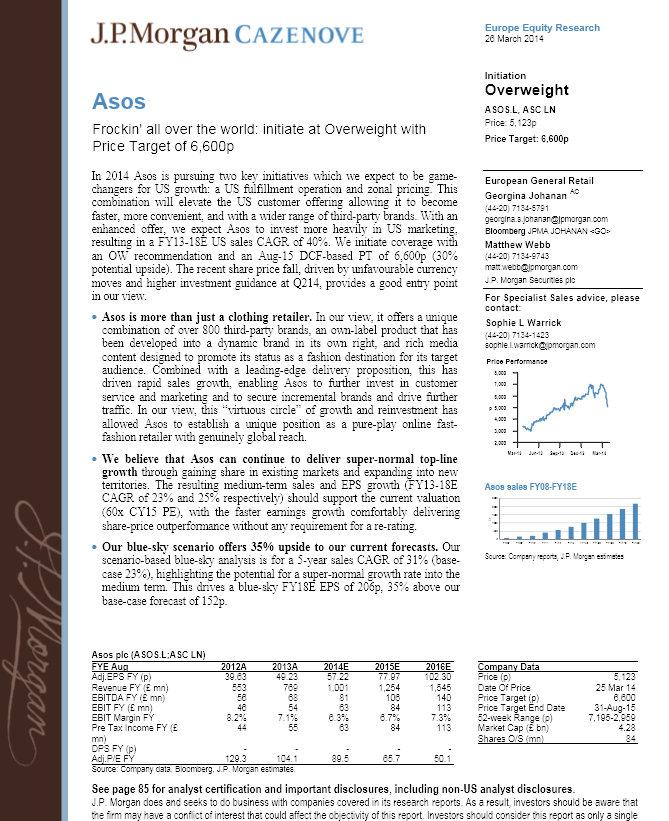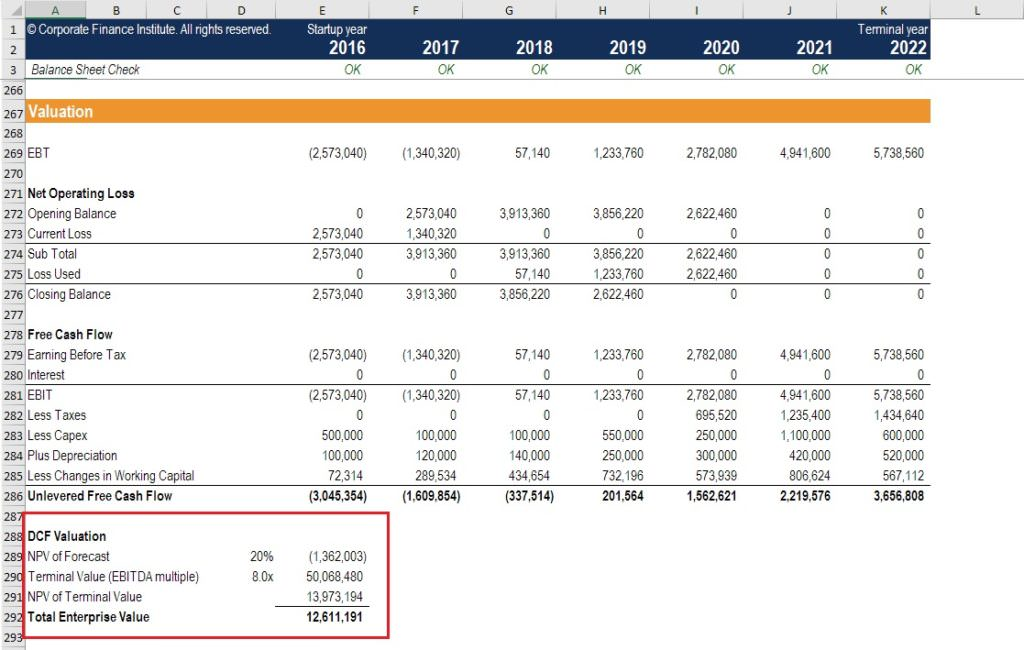# Equity Research Interview Questions

Below are real examples of the most common questions (and answers) used to hire equity research analysts and associates at banks.

## What are the most common equity research interview questions?

Based on our first-hand experience, as well discussions with equity research professionals, we’ve compiled a list of the top questions to be asked by a research analyst when interviewing an associate. We’ve also added what we think are the best answers to these challenging interview questions.  Here are the top equity research interview questions and answers…#### Pitch me a stock (typically will be followed-up with a challenge – e.g., Why has the market not priced this in?)

These are all variants on one of the most common equity research interview questions – pitch me a stock.  Be prepared to pitch three or four stocks – for example, a large cap stock, a small cap stock, and a stock that you would short.  For any company you are going to pitch, make sure that you have read a few analyst reports and know key information about the company.  You must know basic valuation metrics (EV/EBITDA multiples, PE multiples, etc.), key operational statistics, and the names of key members of the management team (e.g., the CEO).  You also must have at least three key points to support your argument.

#### How do you value a stock?

The most common valuation methods are DCF valuation methods and relative valuation methods using comparable public companies (“Comps”) and precedent transactions (“Precedents”).

#### Why might a high tech company have a higher PE than a grocery retailer?

It can also be shown that the Price-Earnings multiple is driven by (1 – g/ROE) / (r – g) where r is the cost of equity, g is the growth rate, and ROE is return on equity.  A high tech company may have a higher PE because growth expectations for the stock are higher.

#### What drives the PB multiple? Or, why may two companies in the same industry have very different PB multiples?

The PB multiple can be shown to be PE x ROE.  It is therefore driven by return on equity and the drivers of the PE multiple.  It can also be shown that the PE multiple is driven by (1 – g/ROE) / (r – g) where r is the cost of equity, g is the growth rate, and ROE is return on equity.

Since the PB multiple is PE x ROE, this means the PB multiple is ( ROE – g ) / (r – g).  If we assume a zero growth rate, the equation implies that the market value of equity should be equal to the book value of equity if ROE = r.  The PB multiple will be higher than 1 if a company delivers ROE higher than the cost of equity (r).

#### Tell me when you would see a company with a high EV/EBITDA multiple but a low PE multiple.

This relationship implies a significant difference between the firm’s enterprise value and its equity value.  The difference between the two is “net debt”.  As a result, a company with a significant amount of net debt will likely have a higher EV/EBITDA multiple.

#### What is a beta?

Beta is a measure of market (systematic) risk. Beta is used in the capital asset pricing model (CAPM) to determine a cost of equity. Beta measures a stock’s volatility of returns relative to an index. So a beta of 1 has the same volatility of returns as the index, and a beta higher than 1 is more volatile.

#### Why do you unlever beta?

When you look up beta on Bloomberg, it’s levered to reflect the debt of each company. But each company’s capital structure is different and we want to look at how “risky” a company is regardless of what percentage of debt or equity it has. To get that, we need to unlever beta each time. You look up the beta for a group of comparable companies, unlever each one, take the median of the set, and then lever it based on your company’s capital structure. Then you use this Levered Beta in the Cost of Equity calculation. For your reference, the formulas for unlevering and re-levering Beta are below:

Unlevered Beta = Levered Beta / (1 + ((1 – Tax Rate) x (Total Debt/Equity)))
Levered Beta = Unlevered Beta x (1 + ((1 – Tax Rate) x (Total Debt/Equity)))

#### What’s the difference between enterprise value and equity value?

This question is commonly asked in banking, but could easily be one of the frequently asked equity research interview questions as well.  Enterprise value is the value of the company that is attributable to all investors.  Equity value only represents the portion of the company belonging to shareholders.  Enterprise value incorporates the market value of the equity plus the market value of net debt (as well as other sources of funding, if used, such as preferred shares, minority interests, etc.).

#### Can a company have an equity value larger than its enterprise value?

Technically, yes.  Enterprise value is the sum of the market value of equity and net debt (gross debt less cash).  If a company has no interest bearing debt but does have cash, then it will lead to a situation where the equity value is greater than the enterprise value.

#### What are the major valuation methodologies?

• DCF valuation methods
• Relative valuation methods – using comparable public companies and precedent transactions
• Break-up valuation methods – looking at the liquidation or break-up value of the business
• Real options valuation methods – rarer
• Here is an overview of all valuation methods

#### When would you not use a DCF valuation methodology?

You would not use a DCF valuation methodology when a company does not have forecastable cash flows.  An example of this would be a start-up company. Below is a screenshot of a DCF model from CFI’s online financial modeling courses.#### What are the most common multiples used to value a company?

This is one of the most common equity research interview questions.  Here are the main types of valuation multiples:

• EV/EBITDA
• EV/EBIT
• P/E
• P/B

#### Why does Warren Buffett prefer EBIT multiples to EBITDA multiples?

EBITDA excludes depreciation and amortization on the basis that they are “non-cash items.”  However, depreciation and amortization also are a measure of what the company is spending or needs to spend on capital expenditure.  Warren Buffett is credited as having said: “Does management think the tooth fairy pays for capital expenditures?” Here is an article on why Buffett does not like EBITDA.

Compare EBIT vs EBITDA.

#### How is valuing a resource company (e.g., oil and gas, a mining company, etc.) different from valuing a standard company?

First, you need to project the prices of commodities and the company’s reserves. Rather than a standard DCF, you use a Net Asset Value (NAV) model. The NAV model is similar, but everything flows from the company’s reserves rather than a simple revenue growth / EBITDA margin projection. You also look at industry-specific multiples such as P / NAV in addition to the standard multiples. Here are more mining valuation methods.

#### Why do DCF projections typically go out between 5 and 10 years?

The forecast period is driven by the ability to reasonably predict the future.  Less than 5 years is often too short to be useful.  More than 10 years becomes difficult to forecast reliably.

#### What do you use for the discount rate in a DCF valuation?

If you are forecasting free cash flows to the firm, then you normally use the Weighted Average Cost of Capital (WACC) as the discount rate.  If you are forecasting free cash flows to equity, then you use the cost of equity.

#### How do you calculate the terminal value in a DCF valuation?

This is one of the classic equity research interview questions.  Terminal values either use an exit multiple or the Gordon Growth (terminal growth rate) method.

#### Explain why we would use the mid-year convention in a DCF valuation?

With standard DCF, there is an assumption that all cash flows occur at the end of the year.  The mid-year convention adjusts for this distortion by making the assumption that all cash flows come mid-way through the year.  Instead of using discount periods of 1 for the first year, 2 for the second year, etc., in the DCF formula, we use 0.5 for the first year, 1.5 for the second year, and so on. For training on financial modeling click here.

### More interview questions

We hope this has been a helpful guide to equity research interview questions and answers!  If you want more practice, take a look at our other interview guides and interactive career map to advance your finance career:

• FP&A interview questions
• Investment banking interviews
• Credit analyst Q&A
• Accounting interviews
• Behavioral questions

### Financial Analyst Certification

Become a certified Financial Modeling and Valuation Analyst (FMVA)® by completing CFI’s online financial modeling classes and training program!